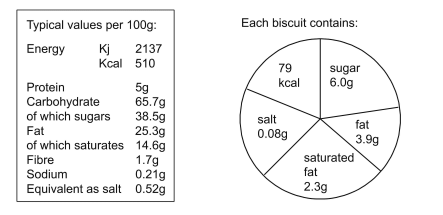# IMAT 2012 Q37 [Biscuits]

The following information appears on a 200g packet of biscuits:How many biscuits are there in a full packet?

A. 22
B. 11
C. 6
D. 13
E. 7

We can look at any of the information from the “Each biscuit contains” and calculate the number of biscuits. I will be looking at the number of Kcal.

Typical values of Kcal in the 200g packet of biscuits can be calculated by multiplying the typical values per 100g by 2, giving us the Kcal in 200g packet as 1020.

To calculate how many biscuits is in the packet we will simply divide the Kcal in the 200g packet by the Kcal of each biscuit:
Number_of_biscuits = \frac{1020}{79} = 12.911 \sim 13 biscuits.

p.s. To get a more precise number, use information that has more precision, like how many salt is there, or saturated fat, or other.